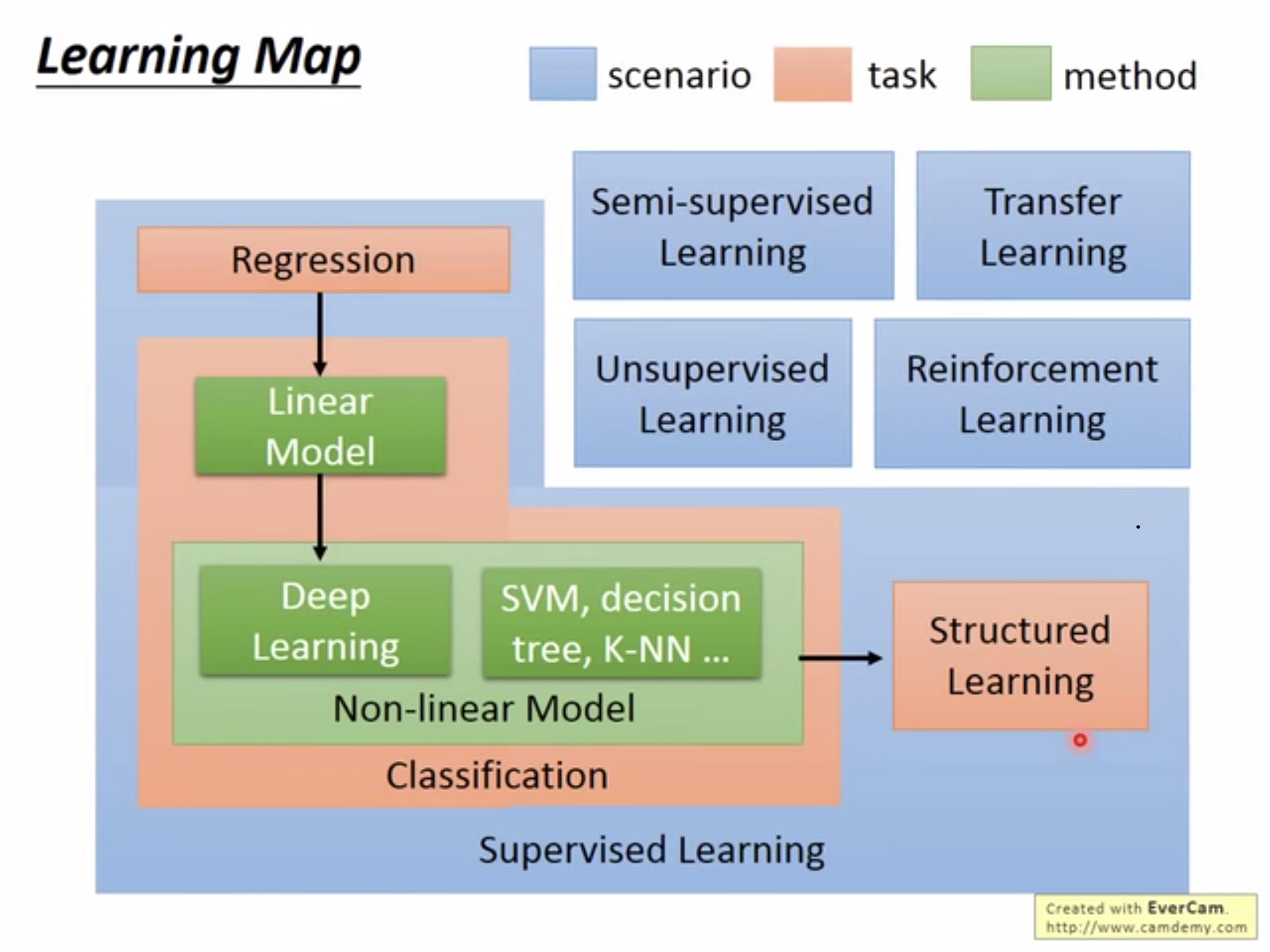# 机器学习笔记P1(李宏毅2019)Regression(回归):输出的目标是一个数值。如预测明天的PM2.5数值。

## (Regression)回归

$L(w, b) = \sum_{i=1}^{i=n}{(\hat y^i-(b+w*x^i))^2}$

$w^{k+1} =w^k - \eta \frac{dL}{dw}|_{w=w^k}$

$L(w, b) = \sum_{k=1}^{n}(\hat y^k - (b + w*x^k))^2$

$\frac{dL}{dw} = 2\sum_{k=1}^{n}(\hat y^k - (b + w*x^k))(-x^k)$
$\frac{dL}{db} = -2\sum_{k=1}{n}{(\hat y^k - (b+w*x^k))}$

$L = \sum_{k=1}^n (\hat y^k- (b + \sum w_kx_k))^2 + \lambda \sum(w_k)^2$

posted @ 2019-11-05 23:38  范中豪  阅读(...)  评论(...编辑  收藏